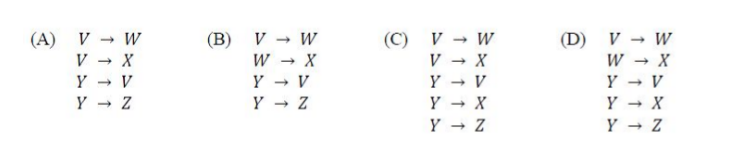Open In App

# GATE | GATE-CS-2017 (Set 1) | Question 15

The following functional dependencies hold true for the relational schema R{V, W, X, Y, Z}:

```V -> W
VW -> X
Y -> VX
Y -> Z
```

Which of the following is irreducible equivalent for this set of functional dependencies?(A) A
(B) B
(C) C
(D) D

Explanation: Given

```V -> W
VW -> X
Y -> VX
Y -> Z```

We need to find the minimal cover of these FDs

Option B. W->X can  not implied by the given FDs, so incorrect

Option C. Y->X can be implied from Y->V and V->X, hence redundant

Option D. W->X can  not implied by the given FDs, so incorrect

Option A. Minimal cover of dependencies that can be extracted out as

1. V -> W
2. V -> X
3. Y -> V
4. Y -> Z

Therefore, option A is most appropriate.

Alternate Solution

Irreducible equivalent functional dependency is minimal cover.
Given,

```  {V → W, VW → X, Y → VX, Y → Z}
```

W extraneous from VW, since we have V → W,

```= {V → W, V → X, Y → VX, Y → Z}
```

X extraneous from VX, since we have V → X,

```=  {V → W, V → X, Y → V, Y → Z}
```

This explanation is contributed by Mithlesh Upadhyay

Quiz of this Question